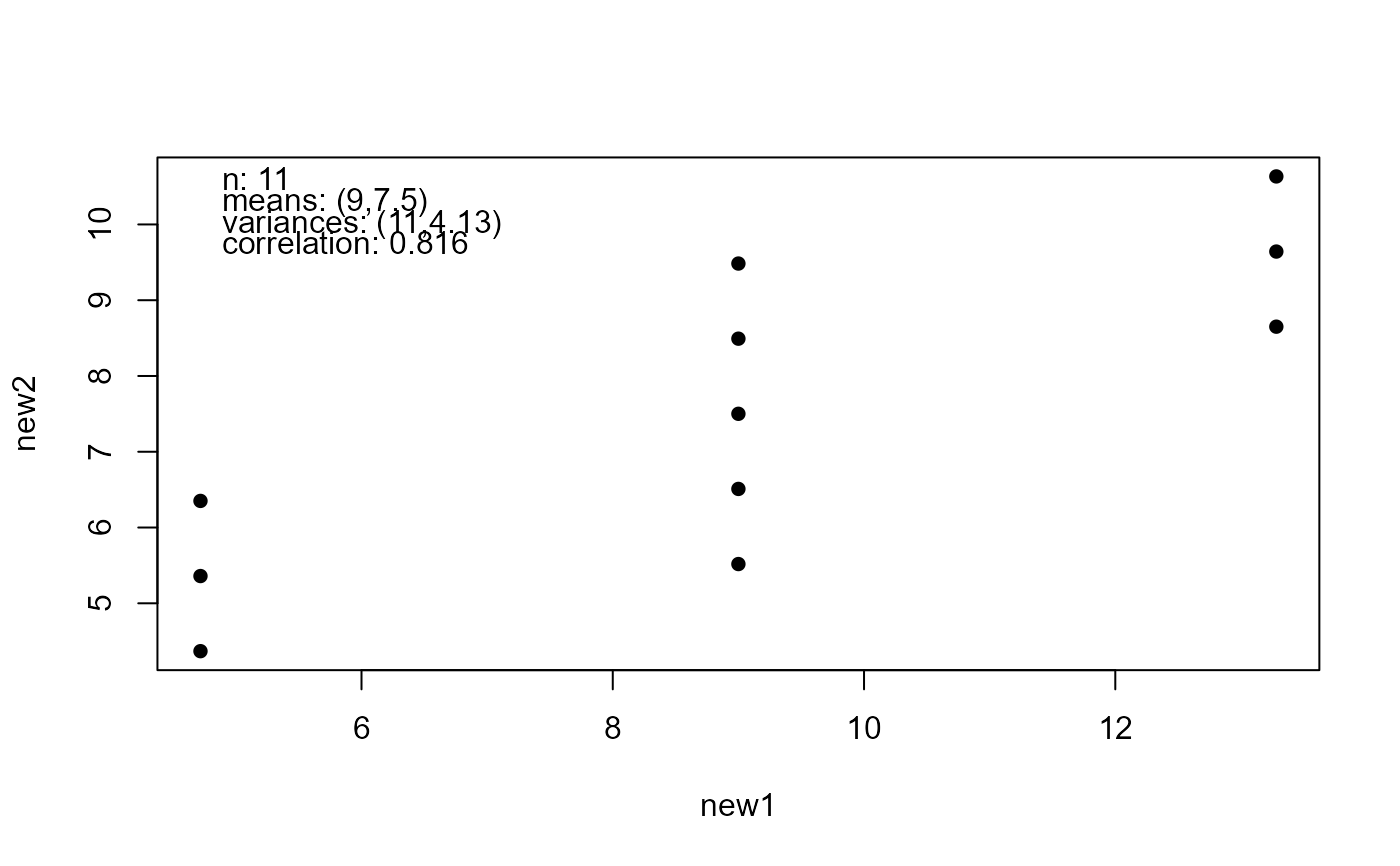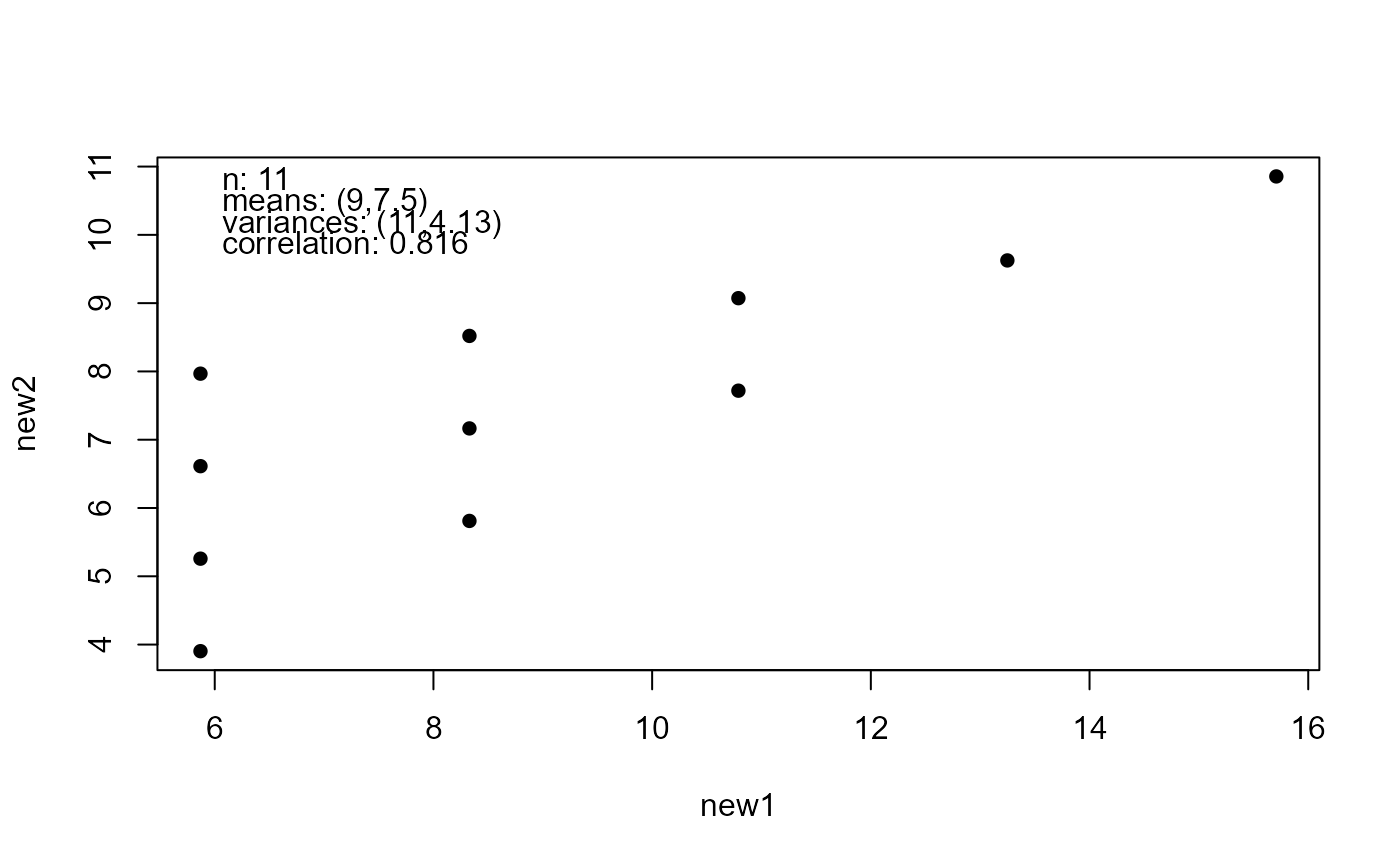Modifies a dataset x so that it shares sample summary statistics with Anscombe's quartet.

anscombise(x, which = 1)

## Arguments

x A numeric matrix or data frame. Each column contains observations on a different variable. Missing observations are not allowed. An integer in {1, 2, 3, 4}. Which of Anscombe's dataset to use. Obviously, this makes very little difference.

## Value

An object of class c("anscombe", class(x)). A dataset with the same format as x. The returned dataset has the following summary statistics in common with Anscombe's quartet.

• The sample means of each variable.

• The sample variances of each variable.

• The sample correlation matrix.

• The estimated regression coefficients from least squares linear regressions of each variable on each other variable. The target and new summary statistics are returned as attributes old_stats and new_stats and the chosen Anscombe's quartet dataset as an attribute old_data.

## Details

The input dataset x is modified by shifting, scaling and rotating it so that its sample mean and covariance matrix match those of the Anscombe quartet.

## See also

mimic to modify a dataset to share sample summary statistics with another dataset.

## Examples

# Old faithful to new faithful
new_faithful <- anscombise(datasets::faithful, which = 4)
plot(new_faithful)# Then check that the sample summary statistics are the same
plot(new_faithful, input = TRUE)# Map of Italy
got_maps <- requireNamespace("maps", quietly = TRUE)
if (got_maps) {
italy <- mapdata("Italy")
new_italy <- anscombise(italy, which = 4)
plot(new_italy)
}